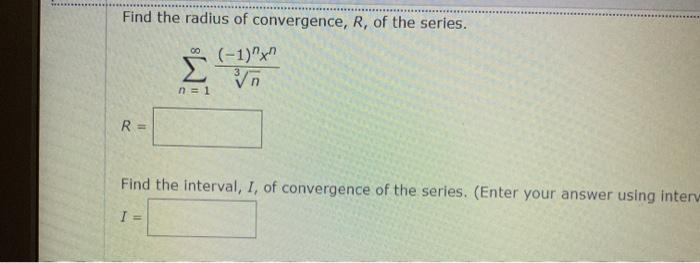### Create an Account

Home / Questions / Find the radius of convergence, R, of the series. 00 (-1) Σ n=1 R= Find the interval, I, o...

# Find the radius of convergence, R, of the series. 00 (-1) Σ n=1 R= Find the interval, I, of convergence of the series. (Enter your answer using interv I =

Find the radius of convergence, R, of the series. 00 (-1) Σ n=1 R= Find the interval, I, of convergence of the series. (Enter your answer using interv I =Jun 03 2021 View more View LessSubscribe To Get Solution